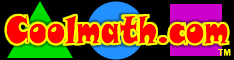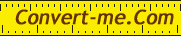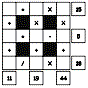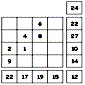Math Resources on the Internet

Silver Burdett Ginn Math Exercises

All grade levels.  These lessons coordinate with the chapters in the SBG math textbooks.

SBG Activity and Answer Sheets - for the lessons above - grade 3, 4, 5, and 6.

Harcourt Activities for Parents and Teachers

Print these cards to practice addition facts.

This printable game provides an entertaining way to practice basic multiplication facts.

• The One-Hundred Mile Sled Race (Grade 6)
Practice adding and subtracting mixed numbers while playing this game.

Harcourt Math Resources and Activities for Students

• Animated Math Glossary
A great reference for math terms and definitions

Drag the sum of 2 numbers onto the addition board.

• Willy the Watchdog - Grade 2
Work with Willy to tell time - but even if you move the hands of the clock incorrectly, you still move around the board.

• Math Matching - Grade 3
Match the fractions to the models.

• Multiplication Mystery - Grade 4
Find the missing factors to solve the mystery!  Drag the product of 2 factors onto multiplication a board.

• Math Matching - Grade 5
Match the equivalent customary measures.

• Fraction Man - Grade 6
Launch fabulous fireworks with Fraction Man!

A+ Math Flashcards
These flashcard games cover the 4 basic operations, rounding, and square roots.
You can also print out your own flashcards.

A+ Math Worksheets
These worksheets can be done and scored online, or you can print them out.
Many areas of math are covered, the 4 basic operations, fractions, decimals, counting money, order of operations, and basic algebra.

A+ Games
Matho (timed math and bingo), hidden picture games, and concentration.

Math Flash
Interactive practice with 4 basic operations.  You pick the operation and the level of difficulty.

Cool Math 4 Kids
Lessons, games, and fun stuff for all ages.Rick's Math Web

Get a piece of graph paper from Rick.

IDEAS:  Copy and paste Graph Paper #2 into Paint to create an Indian-style design, a repeating pattern, or a line or bar graph.

Or click here to go directly to another piece of graph paper.Interactive units converter

Funbrain Math Baseball Game

Funbrain Change Maker Game

Count up to make the correct change.

Funbrain Number Cracker

Figure the next number in a series.

Funbrain Soccer Shootout

Fractions and whole numbers

Funbrain Tic Tac Toe Math

Play against the computer.

Funbrain Line Jumper

Click on points on a number line.  Pick level of difficulty.

Funbrain Guess the Number

Try to guess a number based on too low--too high hints.

Funbrain Guess the Number Plus

Same stuff, just harder.

Practice spelling number words.

Funbrain Fresh Baked Fractions

Click on the fraction that is not equal to the others.

Funbrain Math Car Racing

Funbrain Power Football

Funbrain What's the Point?

Practice with graphing skills

Funbrain Measure It

Practice with measuring in centimeters and inches.

Funbrain Penguin Waiter

Practice calculating the correct per cent tip.

Funbrain Shape Surveyor

Practice with area and perimeter.

Funbrain Place Value Puzzler

Practice with place value and rounding.Discovery School Puzzlemaker Math Square

Math formulas are linked together to form a square.Discovery School Puzzlemaker Number Block

Creates a block of numbers that will challenge arithmetic and algebra skills.
Each row, column and diagonal add up to a total but some numbers are missing.

Discovery School Web Math

Click on a problem solver, enter your math problem and click for an explanation of how it's done and the answer.

Discovery School Web Math Story Problems - Grades 3-8

Me and My Math

Numbers, place value, basic operations, fractions, number lines and laws of arithmetic---with quizzes!